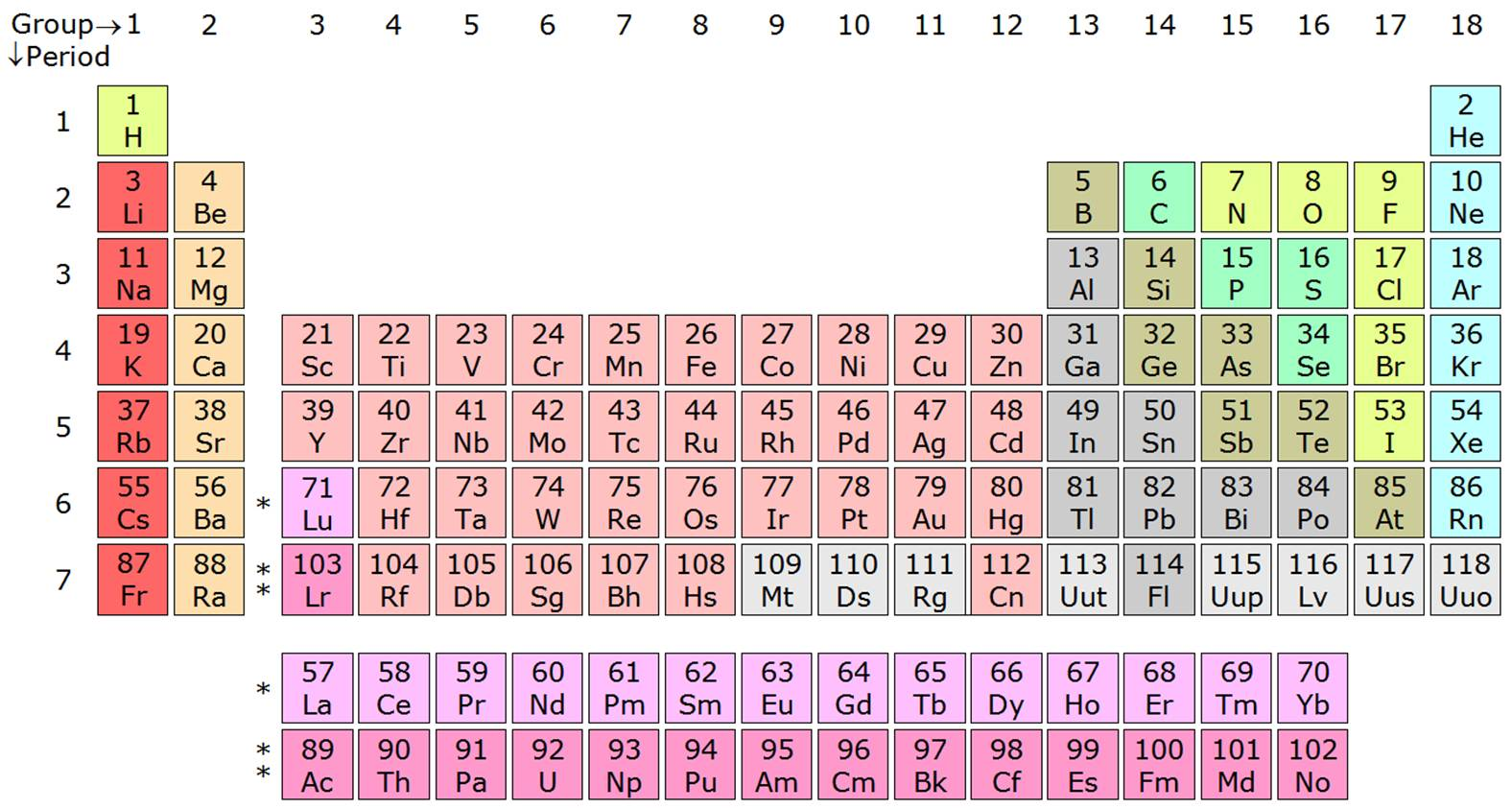basic chemistry PRE-TEST 2018
Last Name *
First Name *
period *
1. Which of the following is the most important factor in determining an element’s place in the periodic table?
0 points
2. Oxygen has an atomic number of 8. Which of the following elements would you expect to be most similar to oxygen in terms of its chemical properties?
1 point
3. Potassium (K), atomic number 19, will most likely react with which of the following elements?
1 point
4. In order to be identified as the element carbon (C), an atom must have ______.
1 point
5. Group 1 (the alkali metals) includes lithium (Li), sodium (Na), and potassium (K). These elements have similar chemical properties because they have the same __________.
1 point
6. The chemical properties of an element are determined by its:
1 point
7. The atomic number of iron is 26, and the atomic mass is 55.847. What do these numbers mean in regard to protons, electrons and neutrons?
1 point
8. Study the table below (table 1). Which element (i.e., atom) has a net positive (+) charge?
1 point
table 1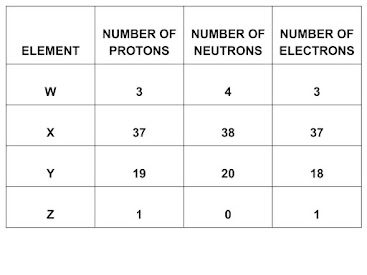9. Refer to diagram 2, Below. Which element in this group would be the least likely to react with other elements?
1 point
Diagram 2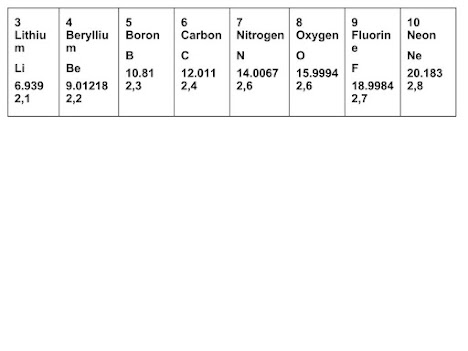10. The graph (graph 1) below shows the percent by mass of six common elements in the human body. Element 6 is most likely
1 point
graph 1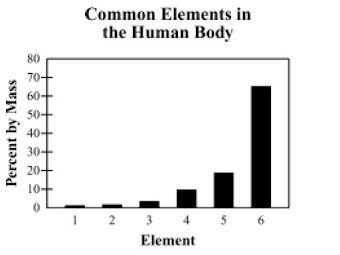11. Carbohydrates, lipids, proteins, and nucleic acids are organic molecules essential for life. Which of the following elements makes the large structures of these organic molecules possible?
1 point
12. Cellulose is a complex carbohydrate that makes up the cell walls of plants. Which of the following elements are main components of cellulose?
0 points
13. Which of the following identifies the number and location of protons in a lithium atom?
1 point
14. A sample of vinegar has a pH of 5, and a sample of rainwater has a pH of 6. Which of the following statements best describes these two substances?
0 points
15. Which of the following statements describes what happens in a buffer solution when a strong acid is added?
0 points
16. Based on valence electron configurations, which of the following is a formula for a compound formed from sodium (Na) and oxygen (O)?
1 point
17. In question 16 above, what type of bond is "holding" the molecule sodium oxide together?
1 point
18. Electrons are transferred from one element to another in a…
1 point
19. Which of the following lewis structures are correct? see diagram 3 below
1 point
diagram 3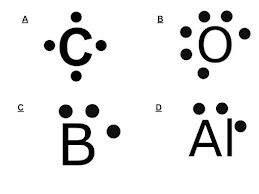20. Carbon has 4 valence electrons. As a result, it forms _________, covalent bonds with other atoms.
1 point
21. Below is the structural formula for methane. (diagram 4). What type of bonds are "holding" this molecule together?
1 point
diagram 4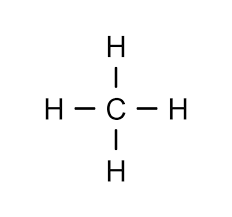22. In diagram 4 above, what would be it's proper molecular formula?
1 point
Periodic Table